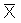## Estimators

An estimator is a statistic which is used to estimate a parameter.

Probability distributions depend upon parameters. For example, the normal distribution depends upon the parameters m and s2 (the mean and variance). In some situations, these parameters may be unknown and we may wish to estimate them. An estimator is a statistic which is used to estimate a parameter.

Desirable Characteristics

Good estimators are those which have a small variance and small bias. The bias of an estimator q which is estimating a parameter p is E(q) - p .

An estimator is unbiased if the bias is zero. The sample mean and sample variance are unbiased estimators of the mean and variance.

So the best estimator for a particular parameter is that for which B(q) + V(q) is smallest, where B(q) is the bias of q.

Example

X1, X2, ..., Xn is a random sample taken from a normal distribution with mean m and variance s2, where m is unknown. Show that the sample mean is an unbiased estimator for m.

We calculated that the expectation of the sample mean is m. Hence E() - m = 0 . So the sample mean is unbiased.

NB: Var() = s2/n , so as n gets large, Var() gets closer to zero and so the sample mean,, has a small variance for large values of n. It turns out thatis the best estimator for m.